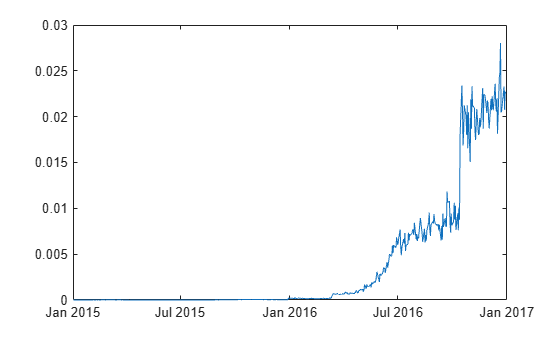Main Content

# mertonByTimeSeries

Estimate default probability using time-series version of Merton model

## Syntax

``````[PD,DD,A,Sa] = mertonByTimeSeries(Equity,Liability,Rate)``````
``````[PD,DD,A,Sa] = mertonByTimeSeries(___,Name,Value)``````

## Description

example

``````[PD,DD,A,Sa] = mertonByTimeSeries(Equity,Liability,Rate)``` estimates the default probability of a firm by using the Merton model.```

example

``````[PD,DD,A,Sa] = mertonByTimeSeries(___,Name,Value)``` adds optional name-value pair arguments. ```

## Examples

collapse all

Load the data from `MertonData.mat`.

```load MertonData.mat Dates = MertonDataTS.Dates; Equity = MertonDataTS.Equity; Liability = MertonDataTS.Liability; Rate = MertonDataTS.Rate;```

Compute the default probability by using the time-series approach of Merton's model.

```[PD,DD,A,Sa] = mertonByTimeSeries(Equity,Liability,Rate); plot(Dates,PD)```Load the data from `MertonData.mat`.

```load MertonData.mat Dates = MertonDataTS.Dates; Equity = MertonDataTS.Equity; Liability = MertonDataTS.Liability; Rate = MertonDataTS.Rate;```

Compute the plot for the default probability values by using the time-series approach of Merton's model. You compute the `PD0` (blue line) by using the default values. You compute the `PD1` (red line) by specifying an optional `Drift` value.

```PD0 = mertonByTimeSeries(Equity,Liability,Rate); PD1 = mertonByTimeSeries(Equity,Liability,Rate,'Drift',0.10); plot(Dates, PD0, Dates, PD1)```## Input Arguments

collapse all

Market value of the firm’s equity, specified as a positive value.

Data Types: `double`

Liability threshold of the firm, specified as a positive value. The liability threshold is often referred to as the default point.

Data Types: `double`

Annualized risk-free interest rate, specified as a numeric value.

Data Types: `double`

### Name-Value Pair Arguments

Specify optional comma-separated pairs of `Name,Value` arguments. `Name` is the argument name and `Value` is the corresponding value. `Name` must appear inside quotes. You can specify several name and value pair arguments in any order as `Name1,Value1,...,NameN,ValueN`.

Example: `[PD,DD,A,Sa] = mertonByTimeSeries(Equity,Liability,Rate,'Maturity',4,'Drift',0.22,'Tolerance',1e-5,'NumPeriods',12)`

Time to maturity corresponding to the liability threshold, specified as the comma-separated pair consisting of `'Maturity'` and a positive value.

Data Types: `double`

Annualized drift rate, expected rate of return of the firm's assets, specified as the comma-separated pair consisting of `'Drift'` and a numeric value.

Data Types: `double`

Number of periods per year, specified as the comma-separated pair consisting of `'NumPeriods'` and a positive integer. Typical values are `250` (yearly), `12` (monthly), or `4` (quarterly).

Data Types: `double`

Tolerance for convergence of the solver, specified as the comma-separated pair consisting of `'Tolerance'` and a positive scalar value.

Data Types: `double`

Maximum number of iterations allowed, specified as the comma-separated pair consisting of `'MaxIterations'` and a positive integer.

Data Types: `double`

## Output Arguments

collapse all

Probability of default of the firm at maturity, returned as a numeric.

Distance-to-default, defined as the number of standard deviations between the mean of the asset distribution at maturity and the liability threshold (default point), returned as a numeric.

Value of firm's assets, returned as a numeric value.

Annualized firm's asset volatility, returned as a numeric value.

## More About

collapse all

### Merton Model for Time Series

In the Merton model, the value of a company's equity is treated as a call option on its assets, and the liability is taken as a strike price.

Given a time series of observed equity values and liability thresholds for a company, `mertonByTimeSeries` calibrates corresponding asset values, the volatility of the assets in the sample's time span, and computes the probability of default for each observation. Unlike `mertonmodel`, no equity volatility input is required for the time-series version of the Merton model. You compute the probability of default and distance-to-default by using the formulae in Algorithms.

## Algorithms

Given the time series for equity (E), liability (L), risk-free interest rate (r), asset drift (μA), and maturity (T), `mertonByTimeSeries` sets up the following system of nonlinear equations and solves for a time series asset values (A), and a single asset volatility (σA). At each time period t, where t = `1`...n:

`$\begin{array}{l}{A}_{1}=\left(\frac{{E}_{1}+{L}_{1}{e}^{-{r}_{1}{T}_{1}}N\left({d}_{2}\right)}{N\left({d}_{1}\right)}\right)\\ {A}_{t}=\left(\frac{{E}_{t}+{L}_{t}{e}^{-{r}_{t}{T}_{t}}N\left({d}_{2}\right)}{N\left({d}_{1}\right)}\right)\\ ...\\ {A}_{n}=\left(\frac{{E}_{n}+{L}_{n}{e}^{-{r}_{n}{T}_{n}}N\left({d}_{2}\right)}{N\left({d}_{1}\right)}\right)\end{array}$`

where N is the cumulative normal distribution. To simplify the notation, the time subscript is omitted for d1 and d2. At each time period, d1, and d2 are defined as:

`${d}_{1}=\frac{\mathrm{ln}\left(\frac{A}{L}\right)+\left(r+0.5{\sigma }_{A}^{2}\right)T}{{\sigma }_{A}\sqrt{T}}$`

`${d}_{2}={d}_{1}-{\sigma }_{A}\sqrt{T}$`

The formulae for the distance-to-default (DD) and default probability (PD) at each time period are:

`$DD=\frac{\mathrm{ln}\left(\frac{A}{L}\right)+\left({\mu }_{A}-0.5{\sigma }_{A}^{2}\right)T}{{\sigma }_{A}\sqrt{T}}$`

`$PD=1-N\left(DD\right)$`

 Zielinski, T. Merton's and KMV Models In Credit Risk Management.

 Loeffler, G. and Posch, P.N. Credit Risk Modeling Using Excel and VBA. Wiley Finance, 2011.

 Kim, I.J., Byun, S.J, Hwang, S.Y. An Iterative Method for Implementing Merton.

 Merton, R. C. “On the Pricing of Corporate Debt: The Risk Structure of Interest Rates.” Journal of Finance. Vol. 29. pp. 449–470.

## Support

#### Effective Model Risk Management with MATLAB

Download white paper